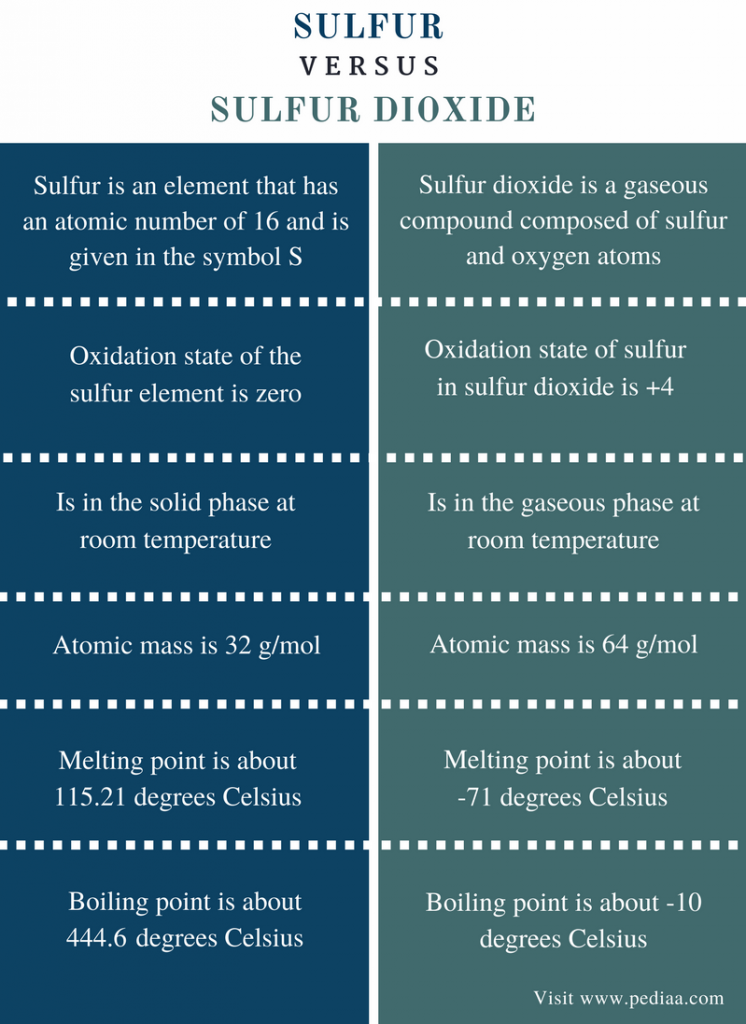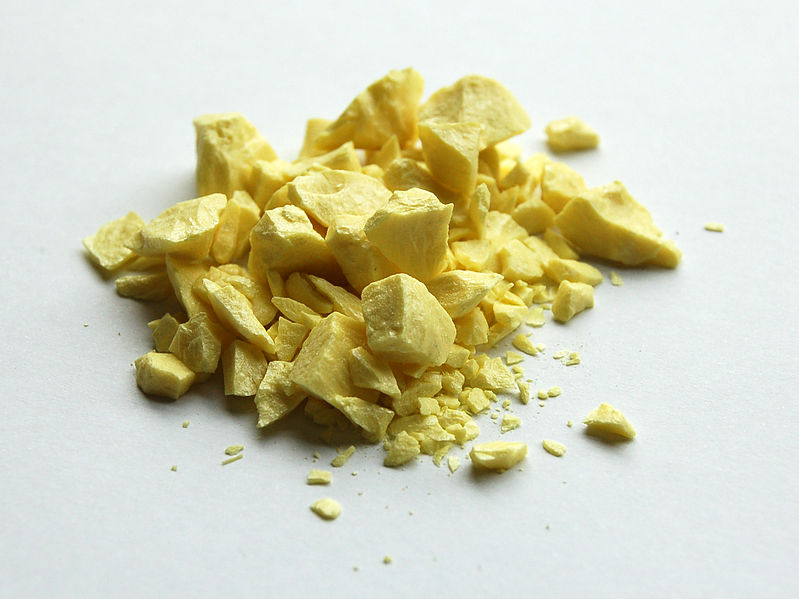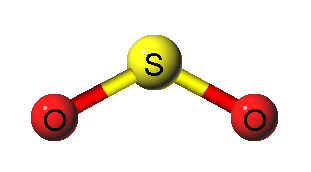# Difference Between Sulfur and Sulfur Dioxide

## Main Difference – Sulfur vs Sulfur Dioxide

Sulfur is a nonmetal that can be found in different molecular forms known as allotropes. It is found in the earth crust as a bright yellow colored solid. Sulfur cannot be found in the atmosphere as a pure element; it is found as oxides of sulfur. The major oxides that can be found in the atmosphere are sulfur dioxide and sulfur trioxides. Sulfur can also be found as the hydride, hydrogen sulfide. The main difference between sulfur and sulfur dioxide is that sulfur is an element whereas sulfur dioxide is a gaseous compound.

### Key Areas Covered

1. What is Sulfur
– Definition, Physical and Chemical Properties, Uses
2. What is Sulfur Dioxide
– Definition, Physical and Chemical Properties, Uses
3. What is the Relationship Between Sulfur and Sulfur Dioxide
– Sulfur and Sulfur Dioxide
4. What is the Difference Between Sulfur and Sulfur Dioxide
– Comparison of Key Differences

Key Terms: Allotropes, Nonmetal, Sulfur, Sulfur Dioxide, Sulfur Trioxide## What is Sulfur

Sulfur is an element that has the atomic number of 16 and is given in the symbol S. This element belongs to the p block of the periodic table and is a nonmetal. The atomic weight of sulfur is about 32 g/mol. The electron configuration can be given as [Ne] 3s23p4. Since it has d orbitals in the 3rd shell, sulfur can have different oxidation states from -2 to +6. Therefore, sulfur can be found in various types of compounds.

At room temperature and pressure, sulfur is a solid. This solid is made out of S8 units. The structure of the S8 unit can occur in different forms. These forms are called allotropes of sulfur. The most common structures of S8 unit are the crown structure and the orthorhombic structure. The melting point of sulfur is 115.21oC, and the boiling point is 444.6oC.Figure 1: Solid Sulfur

Sulfur has about 25 isotopes. The most abundant isotope of sulfur is 32S. The abundance of this isotope on earth is about 94%. Sulfur can be found in the form of sulfides in different types of meteorites. Most of the times, sulfur occurs near hot springs and volcanoes. Theretofore, volcanic deposits can be mined to obtain sulfur element. Sulfur is used to produce all sulfur-containing compounds that are useful in industrial scale as well as laboratory scale.

## What is Sulfur Dioxide

Sulfur dioxide is a gaseous compound composed of sulfur and oxygen atoms. The chemical formula of sulfur dioxide is SO2. Therefore, it is composed of a sulfur atom bonded to two oxygen atoms through covalent bonds. One oxygen atom can form a double bond with the sulfur atom. Hence, the sulfur atom is the central atom of the compound. Since sulfur element has 6 electrons in its outermost orbital, after forming two double bonds with the oxygen atoms, there are 2 more electrons remaining, which can act as a lone electron pair. This determines the geometry of the SO2 molecule as angular geometry.Figure 2: Angular Geometry of Sulfur Dioxide

Sulfur dioxide is considered as a toxic gas. Hence, if there is SO2 in the atmosphere, it will be an indication of air pollution. This gas has a very irritating smell. The molecular mass of sulfur dioxide is 64 g/mol. It is a colorless gas at room temperature. The melting point is about -71oC whereas the boiling point is -10oC.

Sulfur dioxide can be produced by the sulfur combustion process. If not, burning of sulfur containing compounds may also produce sulfur dioxide.

S(s)    +    O2(g)    →    SO2(g)

This reaction is exothermic. Therefore, it releases energy along with sulfur dioxide gas. The heat produced from this energy is very high. Moreover, sulfur containing compounds such as ferrous sulfide, zinc sulfide may release sulfur dioxide gas.

FeS2(s)  +   O2(g)    →   Fe2O3(s)   +    SO2(g)

The oxidation state of sulfur in sulfur dioxide is +4. Therefore, sulfur dioxide can also be produced by the reduction of compounds composed of sulfur atoms that are in a higher oxidation state. One such example is the reaction between copper and sulfuric acid. Here, sulfur in sulfuric acid is in the oxidation state of +6. Therefore, it can be reduced into +4 oxidation state of sulfur dioxide.

Sulfur dioxide can be used in the production of sulfuric acid which has a number of applications in the industrial scale and the laboratory scale. Sulfur dioxide is also a good reducing agent. Since the oxidation state of sulfur is +4 in sulfur dioxide, it can easily be oxidized to +6 oxidation state which allows another compound to be reduced.

## Relationship Between Sulfur and Sulfur Dioxide

• Sulfur dioxide is produced when solid sulfur is burnt in the presence of oxygen.

## Difference Between Sulfur and Sulfur Dioxide

### Definition

Sulfur: Sulfur is an element that has an atomic number of 16 and is given in the symbol S.

Sulfur Dioxide: Sulfur dioxide is a gaseous compound composed of sulfur and oxygen atoms.

### Oxidation State

Sulfur: The oxidation state of the sulfur element is zero.

Sulfur Dioxide: The oxidation state of sulfur in sulfur dioxide is +4.

### Phase

Sulfur: Sulfur is in the solid phase at room temperature.

Sulfur Dioxide: Sulfur dioxide is in the gaseous phase at room temperature.

### Mass

Sulfur: The atomic mass of sulfur is 32 g/mol.

Sulfur Dioxide: The molecular mass of sulfur dioxide is 64 g/mol.

### Melting Point

Sulfur: The melting point of sulfur is about 115.21oC.

Sulfur Dioxide: The melting point of sulfur dioxide is about -71oC.

### Boiling Point

Sulfur: The boiling point of sulfur is about 444.6oC.

Sulfur Dioxide: The boiling point of sulfur dioxide is about -10oC.

### Conclusion

Sulfur forms two main oxides that are gases at room temperature. They are sulfur dioxide and sulfur monoxide. Sulfur dioxide can be produced from the combustion of sulfur. Although sulfur dioxide is also composed of sulfur atoms, they exhibit different chemical and physical properties. The main difference between sulfur and sulfur dioxide is that sulfur is an element whereas sulfur dioxide is a gaseous compound.

##### References:

1. “Sulfur dioxide.” Wikipedia. Wikimedia Foundation, 05 Aug. 2017. Web. Available here. 08 Aug. 2017.
2. “Sulfur dioxide.” Encyclopædia Britannica. Encyclopædia Britannica, inc., n.d. Web. Available here. 08 Aug. 2017.

##### Image Courtesy:

1. “Sulfur-sample” By Ben Mills – Own work (Public Domain) via Commons Wikimedia
2. “Sulfur-Dioxide-diagram” The original uploader was Pdefer at English Wikipedia – Transferred from en.wikipedia to Commons by Edgar181 using CommonsHelper. (CC BY-SA 3.0) via Commons Wikimedia